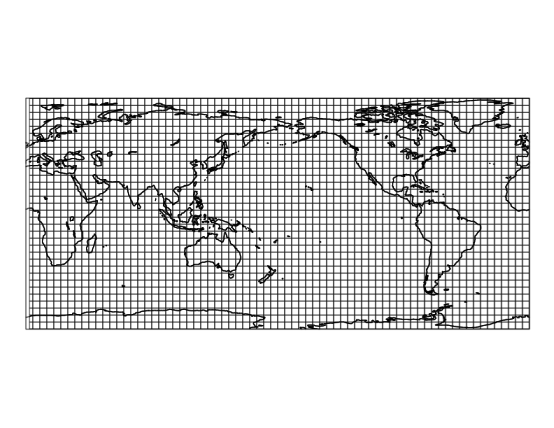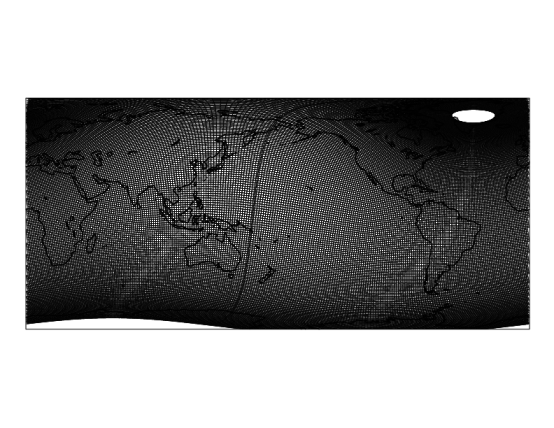# Plot grid and transect with PyNGL and komod

Draw a grid and a transect with PyNGL module



Solution:

komod module



Notebook file

This is a short follow up of the previous post about komod module, that is essentially a set of wrapper functions for PyNGL module. Here I am going to show how to plot a grid of your model and how to draw a transect. I am going to use the same data set af before: mean temperature from the World Ocean Atlas 2009 (5 deg. resolution).

Import modules:

In :
import komod
import Nio
from IPython.display import Image
import numpy as np


Get variables from the netCDF file:

In :
ff =Nio.open_file('temperature_annual_5deg.nc')
temp = ff.variables['t_mn'][:]
lat  = ff.variables['lat'][:]
lon  = ff.variables['lon'][:]
lev  = ff.variables['depth'][:]


Create lat/lon mesh grid:

In :
lon_m, lat_m = np.meshgrid(lon,lat)


Now we can plot our grid. This function use centers of the grid boxes, not corners, so result might not be a perfectly accurate representation of your grid, but rather rough estimate of its shape and density. Only necessary input parameters are 2d arrays of lats and lons:

In :
komod.pltgrd(lon_m,lat_m, region = 'Global', add_cyclic=True)

In :
!convert -scale 70% -rotate -90 grid.ps grid.png
Image(filename='grid.png')

Out:In this case everything is very simple and rectangular. Let's have a look at more interesting case. We will use a grid from one of the MPIOM configurations, and you can download this sample file from here.

In :
g = Nio.open_file('GR15s.nc')
lat_g = g.variables['grid_center_lat'][:]
lon_g = g.variables['grid_center_lon'][:]

In :
komod.pltgrd(lon_g,lat_g, region = 'Global', add_cyclic=True)
!convert -scale 70% -rotate -90 grid.ps grid.png
Image(filename='grid.png')

Out:Very dense grid, you can hardly see any details. We can show only every n$^{th}$ element of grid by specifying every parameter:

In :
komod.pltgrd(lon_g,lat_g, region = 'Global', every=3, add_cyclic=True)
!convert -scale 70% -rotate -90 grid.ps grid.png
Image(filename='grid.png')

Out: